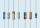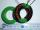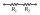Ohm's law - examples

Ohm's law states that the current through a conductor (or resistor) between two points is directly proportional to the voltage across the two points. Introducing the constant of proportionality, the resistance, it arrives at the usual mathematical equation I = U/R or I=V/R

1. ResistorsTwo resistors connected in series give the resulting resistance 216Ω and 48Ω in parallel. Determine the resistance of this resistors.
2. ResistanceDetermine the resistance of the bulb with current 200 mA and is in regular lamp (230V).
3. Coil as a girlThe electrical resistance of the copper wire coil is 2.0 ohms. What current runs through the coil when the voltage between the terminals is 3.0 V?
4. TransformerSolve the textbook problems - transformer: a) N1 = 40, N2 = 80, U2 = 80 V, U1 =? b) N1 = 400, U1 = 200 V, U2 = 50 V, N2 =?
5. The bulbsThe bulbs are connected serially two bulbs and one resistor. Each bulb has a resistivity of 100 ohms and resistor of 60 ohms The voltage source in the circuit is 24 volts. Do you know what voltage to measure on individual appliances?
6. Resistance of the resistorThe resistor terminals have a voltage of 20 V and a current of 5 mA is passed through. What is the resistance of the resistor?
7. Electric input powerSolve problems related to electric power: a) U = 120 V, I = 0.5 A, P =? b) P = 200 W, U = 230 V, I =? c) I = 5 A, P = 2200W, U =?
8. Filament of bulbThe filament of bulb has a 1 ohm resistivity and is connected to a voltage 220 V. How much electric charge will pass through the fiber when the electric current passes for 10 seconds?
9. Two resistorsTwo resistors when they give 25 ohms in series and 4 ohms in parallel what the values of

We apologize, but in this category are not a lot of examples.
Do you have an interesting mathematical example that you can't solve it? Enter it, and we can try to solve it.

To this e-mail address, we will reply solution; solved examples are also published here. Please enter e-mail correctly and check whether you don't have a full mailbox.